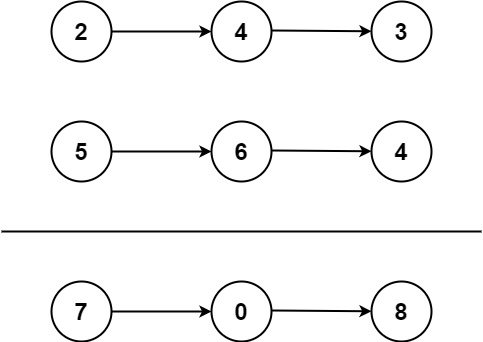You are given two non-empty linked lists representing two non-negative integers. The digits are stored in reverse order, and each of their nodes contains a single digit. Add the two numbers and return the sum as a linked list.

You may assume the two numbers do not contain any leading zero, except the number 0 itself.

Example 1:```Input: l1 = [2,4,3], l2 = [5,6,4]
Output: [7,0,8]
Explanation: 342 + 465 = 807.
```

Example 2:

```Input: l1 = , l2 = 
Output: 
```

Example 3:

```Input: l1 = [9,9,9,9,9,9,9], l2 = [9,9,9,9]
Output: [8,9,9,9,0,0,0,1]
```

Constraints:

• The number of nodes in each linked list is in the range `[1, 100]`.
• `0 <= Node.val <= 9`
• It is guaranteed that the list represents a number that does not have leading zeros.

## Rust Solution

``````struct Solution;
use rustgym_util::*;

impl Solution {
let mut sum: ListLink = None;
let mut p1: &ListLink = &l1;
let mut p2: &ListLink = &l2;
let mut p3: &mut ListLink = &mut sum;
let mut carry = 0;
while p1.is_some() || p2.is_some() || carry != 0 {
let mut val = carry;
if let Some(n1) = p1.as_ref() {
val += n1.val;
p1 = &n1.next;
}
if let Some(n2) = p2.as_ref() {
val += n2.val;
p2 = &n2.next;
}
carry = val / 10;
p3 = &mut p3.as_mut().unwrap().next;
}
sum
}
}

#[test]
fn test() {
let l1 = list!(2, 4, 3);
let l2 = list!(5, 6, 4);
let l3 = list!(7, 0, 8);